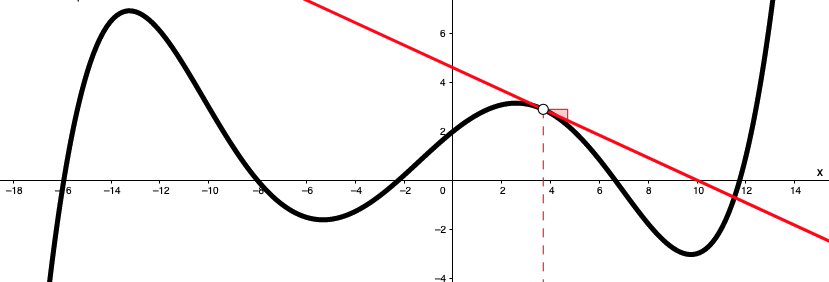# TensorFlow2: Training Loop.## Context

Although Keras is suitable for the vast majority of use cases, in the following scenarios, it may make sense to forgo model.fit() to manually define a training loop:

• Maintaining legacy code and retraining old models.

• Custom batch/ epoch operations like gradients and backpropagation. Even then, PyTorch may be a better fit for customization.

Disclaimer; This notebook demonstrates how to manually define a training loop for queued tuning of a binary classification model. However, it is only included to prove that AIQC technically supports TensorFlow out-of-the-box with analysis_type='keras', and to demonstrate how expert practicioners to do continue to use their favorite tools. We neither claim to be experts on the inner-workings of TensorFlow, nor do we intend to troubleshoot advanced methodologies for users that are in over their heads.

Reference this repository for more TensorFlow cookbooks: > https://github.com/IvanBongiorni/TensorFlow2.0_Notebooks

## 💾 Data

:

from aiqc import datum
df = datum.to_df('sonar.csv')

:

a b c d e f g h i j ... az ba bb bc bd be bf bg bh object
0 0.0200 0.0371 0.0428 0.0207 0.0954 0.0986 0.1539 0.1601 0.3109 0.2111 ... 0.0027 0.0065 0.0159 0.0072 0.0167 0.0180 0.0084 0.0090 0.0032 R
1 0.0453 0.0523 0.0843 0.0689 0.1183 0.2583 0.2156 0.3481 0.3337 0.2872 ... 0.0084 0.0089 0.0048 0.0094 0.0191 0.0140 0.0049 0.0052 0.0044 R
2 0.0262 0.0582 0.1099 0.1083 0.0974 0.2280 0.2431 0.3771 0.5598 0.6194 ... 0.0232 0.0166 0.0095 0.0180 0.0244 0.0316 0.0164 0.0095 0.0078 R
3 0.0100 0.0171 0.0623 0.0205 0.0205 0.0368 0.1098 0.1276 0.0598 0.1264 ... 0.0121 0.0036 0.0150 0.0085 0.0073 0.0050 0.0044 0.0040 0.0117 R
4 0.0762 0.0666 0.0481 0.0394 0.0590 0.0649 0.1209 0.2467 0.3564 0.4459 ... 0.0031 0.0054 0.0105 0.0110 0.0015 0.0072 0.0048 0.0107 0.0094 R

5 rows × 61 columns

:

from aiqc.orm import Dataset
shared_dataset = Dataset.Tabular.from_df(df)


## 🚰 Pipeline

:

from aiqc.mlops import Pipeline, Input, Target, Stratifier
from sklearn.preprocessing import LabelBinarizer, PowerTransformer

:

pipeline = Pipeline(
Input(
dataset  = shared_dataset,
encoders = Input.Encoder(
PowerTransformer(method='yeo-johnson', copy=False),
dtypes = ['float64']
)
),

Target(
dataset = shared_dataset,
column  = 'object',
encoder = Target.Encoder(LabelBinarizer(sparse_output=False))
),

Stratifier(
size_test       = 0.12,
size_validation = 0.22
)
)


## 🧪 Experiment

:

from aiqc.mlops import Experiment, Architecture, Trainer
from aiqc.utils.tensorflow import batcher
import tensorflow as tf
from tensorflow.keras import layers as l

:

def fn_build(features_shape, label_shape, **hp):
m = tf.keras.models.Sequential(name='Sonar')
return m

:

def fn_lose(**hp):
loser = tf.losses.BinaryCrossentropy()
return loser

:

def fn_optimize(**hp):
return optimizer

:

def fn_train(
model, loser, optimizer,
train_features, train_label,
eval_features, eval_label,
**hp
):
batched_train_features, batched_train_labels = batcher(
train_features, train_label, batch_size = 5
)

# Still necessary for saving entire model.
model.compile(loss=loser, optimizer=optimizer)

## --- Metrics ---
acc = tf.metrics.BinaryAccuracy()
# Mirrors keras.model.History.history object.
history = {
'loss':list(), 'accuracy': list(),
'val_loss':list(), 'val_accuracy':list()
}

## --- Training loop ---
for epoch in range(hp['epochs']):
# --- Batch training ---
for i, batch in enumerate(batched_train_features):

batch_loss = loser(
batched_train_labels[i],
model(batched_train_features[i])
)
# Update weights based on the gradient of the loss function.

## --- Epoch metrics ---
# Overall performance on training data.
train_probability = model.predict(train_features)
train_loss = loser(train_label, train_probability)
train_acc = acc(train_label, train_probability)
history['loss'].append(float(train_loss))
history['accuracy'].append(float(train_acc))
# Performance on evaluation data.
eval_probability = model.predict(eval_features)
eval_loss = loser(eval_label, eval_probability)
eval_acc = acc(eval_label, eval_probability)
history['val_loss'].append(float(eval_loss))
history['val_accuracy'].append(float(eval_acc))
# Attach history to the model so we can return a single object.
model.history.history = history
return model

:

hyperparameters = dict(
neuron_count = [25, 50], epochs = [25, 50]
)

:

experiment = Experiment(
Architecture(
library           = "keras"
, analysis_type   = "classification_binary"
, fn_build        = fn_build
, fn_train        = fn_train
, fn_lose         = fn_lose
, fn_optimize     = fn_optimize
, hyperparameters = hyperparameters
),

Trainer(pipeline=pipeline)
)

:

experiment.run_jobs()

📦 Caching Splits 📦: 100%|██████████████████████████████████████████| 3/3 [00:00<00:00, 368.00it/s]
🔮 Training Models 🔮: 100%|██████████████████████████████████████████| 4/4 [01:21<00:00, 20.41s/it]


## 📊 Visualization & Interpretation

For more information on visualization of performance metrics, reference the Dashboard documentation.